### Home > CALC > Chapter 4 > Lesson 4.5.1 > Problem4-164

4-164.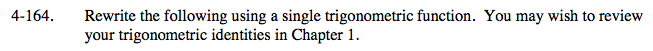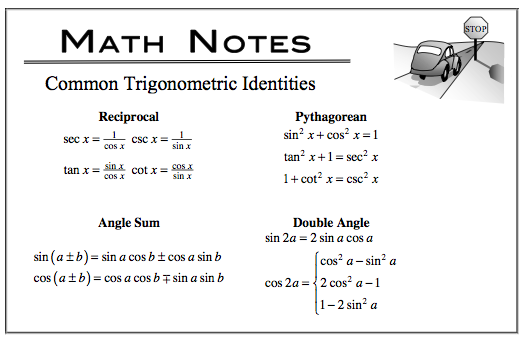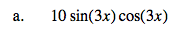Double Angle identity (factor first).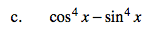Factor first.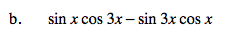Sum and Difference (Angle Sum) identity.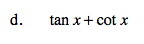Start by rewriting tan(x) and cot(x) as fractions.

You will use more than one identity.

$\frac{\text{sin}x}{\text{cos}x}+\frac{\text{cos}x}{\text{sin}x}=\frac{\text{sin}^{2}x+\text{cos}^{2}x}{(\text{cos}x)(\text{sin}x)}=$

$\frac{1}{\frac{1}{2}\text{sin}(2x)}=2\text{csc}(2x)$